Courses

# Multiple Correct MCQ Of Ray And Wave Optics, Past Year Questions JEE Advance, Class 12, Physics

## 25 Questions MCQ Test Class 12 Physics 35 Years JEE Mains &Advance Past year Paper | Multiple Correct MCQ Of Ray And Wave Optics, Past Year Questions JEE Advance, Class 12, Physics

Description
This mock test of Multiple Correct MCQ Of Ray And Wave Optics, Past Year Questions JEE Advance, Class 12, Physics for JEE helps you for every JEE entrance exam. This contains 25 Multiple Choice Questions for JEE Multiple Correct MCQ Of Ray And Wave Optics, Past Year Questions JEE Advance, Class 12, Physics (mcq) to study with solutions a complete question bank. The solved questions answers in this Multiple Correct MCQ Of Ray And Wave Optics, Past Year Questions JEE Advance, Class 12, Physics quiz give you a good mix of easy questions and tough questions. JEE students definitely take this Multiple Correct MCQ Of Ray And Wave Optics, Past Year Questions JEE Advance, Class 12, Physics exercise for a better result in the exam. You can find other Multiple Correct MCQ Of Ray And Wave Optics, Past Year Questions JEE Advance, Class 12, Physics extra questions, long questions & short questions for JEE on EduRev as well by searching above.
*Multiple options can be correct
QUESTION: 1

### In the Young’ s double slit experiment, the interference pattern is found to have an intensity ratio between the bright and dark fringes as 9. This implies that

Solution: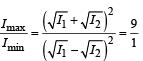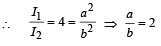*Multiple options can be correct
QUESTION: 2

### A convex lens of focal length 40 cm is in contact with a concave lens of focal length 25 cm . The power of the combination is

Solution: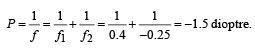*Multiple options can be correct
QUESTION: 3

### White light is used to illuminate the two slits in a Young’s double slit experiment. The separation between the slits is b and the screen is at a distance d (> b) from the slits. At a point on the screen directly in front of one of the slits, certain wavelengths are missing. Some of these missing wavelengths are

Solution: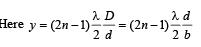(∵ d = b and D = d)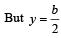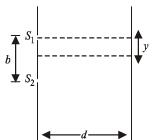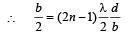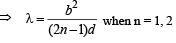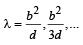*Multiple options can be correct
QUESTION: 4

A converging lens is used to form an image on a screen. When the upper half of the lens is covered by an opaque screen

Solution:

The image formed will be complete because light rays from all parts of the object will strike on the lower half.
But since the upper half light rays are cut off, the intensity will reduce.

*Multiple options can be correct
QUESTION: 5

A short linear object of length b lies along the axis of a concave mirror of focal length f at a distanee u from the pole of the mirror. The size of the image is approximately equal to

Solution: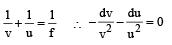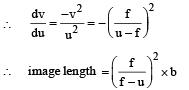*Multiple options can be correct
QUESTION: 6

A beam of light consisting of red, green and blue colours is incident on a right angled prism, fig. The refractive indices of the material of the prism for the above red, green and blue wavelengths are 1.39, 1.44 and 1.47 respectively. The prism will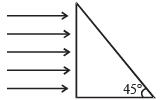Solution:

For total internal reflection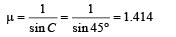i.e. for an angle of incidence of 45°, that colour will suffer total internal reflection for which the refractive index is less than 1.414.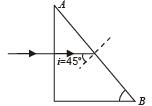Therefore, red light will be refracted at interface AB whereas blue and green light will suffer total internal reflection.

*Multiple options can be correct
QUESTION: 7

An astronomical telescope has an angular magnification of magnitude 5 for distant objects. The separation between the objective and the eyepiece is 36 cm and the final image is formed at infinity. The focal length f0 of the objective and the focal length f0 of the eyepiece are

Solution:

In an astronomical telescope when the object and final image are at infinity, M and L are given as shown: Angular magnification M = fo/fe

Seperation between lenses, L = fo +fe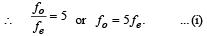fo +fe= 36  or  5 fe +fe= 36

or fe = 6 cm ... (ii)

∴ fo = 5fe  or  fo = 30 cm

Hence fo = 30 cm,  fe = 6 cm

*Multiple options can be correct
QUESTION: 8

A thin prism P1 with angle 4° and made from glass of refractive index 1.54 is combined with another thin prism P2 made from glass of refractive index 1.72 to produce dispersion without deviation. The angle of the prism P2 is

Solution:

The angle of deviation for the first prism P1

δ= (µ1 – 1) A1

The angle of deviation for the second prism P2

δ2 = (µ2 – 1) A2

Since total deviation is to be zero

∴ δ+ δ= 0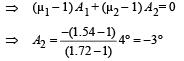*Multiple options can be correct
QUESTION: 9

A planet is observed by an astronomical  refracting telescope having an objective of focal length 16 m and an eyepiece of focal length 2 cm.

Solution:

In case of an astronomical telescope the distance between the objective lens and eyepiece lens

= f0 +fe = 16 + 0.02 = 16.02 m

The angular magnification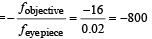NOTE : The image seen by the astronomical telescope is inverted. Also the objective lens is larger than eye piece lens.

*Multiple options can be correct
QUESTION: 10

Two thin convex lenses of focal lengths f1 and f2 are separated by a horizontal distance d (where d <f1, d< f2) and their centres are displaced by a vertical separation Δ as shown in the fig.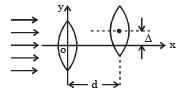Taking the origin of coordinates O, at the centre of the first lens the x and y coordinates of the focal point of this lens system, for a parallel beam of rays coming from the left, are given by:

Solution:

The image I1 of parallel rays formed by lens 1 will act as virtual object.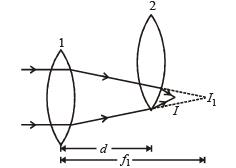Applying lens formula for lens 2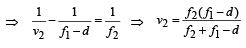∴ The horizontal distance of the image I from O is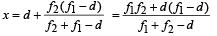To find the y-coordinate, we use magnification formula for lens 2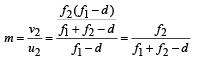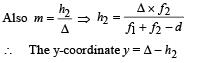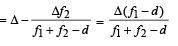*Multiple options can be correct
QUESTION: 11

Which of the following form(s) a virtual and erect image for all positions of the object ?

Solution:

NOTE : Concave lens and convex mirror are diverging in nature. Therefore the refracted/reflected rays do not meet.
These rays are produced backwards to make them  meet.
Therefore the image formed is virtual and erect.

*Multiple options can be correct
QUESTION: 12

A real image of a distant object is formed by a plano-convex lens on its principal axis. Spherical aberration

Solution:

Spherical aberation is smaller when the curved surface is facing the object because the total deviation is shared between the two surfaces.

*Multiple options can be correct
QUESTION: 13

A ray of light travelling in a transparent medium falls on a surface separating the medium from air at an angle of incidence of 45°. The ray undergoes total internal reflection. If n is the refractive index of the medium with respect to air, select the possible value(s) of n from the following :

Solution:

KEY CONCEPT : For total internal reflection to take place :

Angle of incidence i > critical angle, θc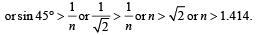Therefore, possible values of n can be 1.5 or1.6 in the given options.

*Multiple options can be correct
QUESTION: 14

A parallel monochromatic beam of light is incident normally on a narrow slit. A diffraction pattern is formed on a screen placed perpendicular to the direction of the incident beam.
At the first minimum of the diffraction pattern, the phase difference between the rays coming from the two edges of the slit is

Solution:

For first minima the path difference between the rays coming from the two edges should be λ which corresponds to a phase difference of 2π.

*Multiple options can be correct
QUESTION: 15

A concave mirror is placed on a horizontal table, with its axis directed vertically upwards. Let O be the pole of the mirror and C its centre of curvature. A point object is placed at C.
It has a real image, also located at C. If the  mirror is now filled with water, the image will be.

Solution:

The ray diagram is shown in  figure. Therefore, the image will be real and between C and O.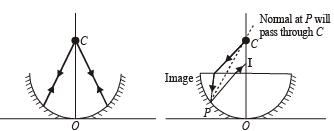*Multiple options can be correct
QUESTION: 16

A spherical surface of radius of curvature R separates air (refractive index 1.0) from glass (refractive index 1.5). The centre of curvature is in the glass. A point object P placed in air is found to have a real image Q in the glass. The line PQ cuts the surface at a point O, and PO = OQ. The distance PO is equal to

Solution:

The formula for spherical refracting surface is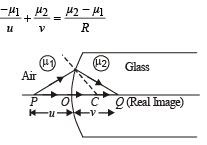Here u = –x, v = + x, R = + R, µ1  = 1, µ2  = 1.5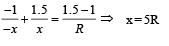*Multiple options can be correct
QUESTION: 17

In a Young’s double slit experiment, the separation between the two slits is d and the wavelength of the light is l. The intensity of light falling on slit 1 is four times the intensity of light falling on slit 2. Choose the correct choice(s).

Solution:

The condition to obtain maxima in the phenomenon observed in young's double slit experiment is d sin θ = nλ where n is an integer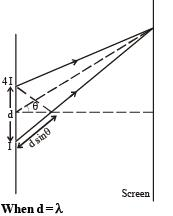l sin q = nλ

⇒ sin q = n

When n = 0, θ = 0
When n = 1, θ = 90° (This will be a point on the screen which will be at infinity and therefore not practical)

Other values of n are invalid  as –1 < sin θ <1 .
⇒ The screen will have only one maxima.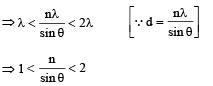The possible values of n are 0,  + 1, –1.
⇒ There is at least one more maxima (besides the central maxima, option [B] is correct.
We know that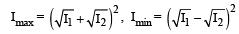Initially I1 = 4 I and I2 = I
∴    Imax = 9 I and Imin = I
When I1 = I2 = I then  Imax  = 4 I and Imin = 0
i.e., when the intensities become equal, Imin reduces to zero.
Options [C] and [D] are incorrect.

*Multiple options can be correct
QUESTION: 18

A student performed the experiment of determination of focal length of a concave mirror by u-v method using an optical bench of length 1.5 meter. The focal length of the mirror used is 24 cm. The maximum error in the location of the image can be 0.2 cm. The 5 sets of (u, v) values recorded by the student  (in cm) are :

(42, 56), (48, 48), (60, 40), (66, 33), (78, 39). The data set(s) that cannot come from experiment and is (are) incorrectly recorded, is (are)

Solution:

Given    f = – 24 cm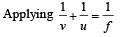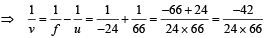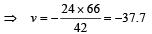But the value of v = 33. The absolute error is 37.7 – 33 = 4.7 cm which is greater than 0.2 cm. Therefore a wrong reading.

For (78, 39) when u = 78 then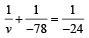⇒   v = – 34.67

The absoluate error is 39 – 34.67 = 4.33 which is greater than 0.2 cm.

*Multiple options can be correct
QUESTION: 19

A ray OP of monochromatic light is incident on the face AB of prism ABCD near vertex B at an incident angle of 60° (see figure). If the refractive index of the material of the prism is √3, which of the following is (are) correct?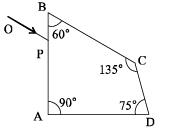Solution:

Applying Snell’s law at P

n1 sin 60° = n2 sinθ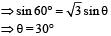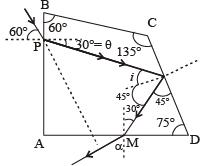60° + (90° + 30°) + 135° + ∠PQC = 360°
⇒ ∠PQC = 45° ⇒  i = 45°

The critical angle for prism - air pair of media is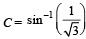which is less than 45°.
Therefore total internal reflection takes place at face CD.
option (a) is correct.
In ΔQDM, ∠QMD = 180° – (45° + 75°) = 60°

Therefore the angle of incidence of ray QM on AD is 30°.
This angle is less than the critical angle. Therefore the ray emerges out of face AD.
Option (b) is correct.
Applying Snell’s law at M, we get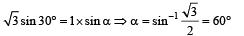In quadrilateral PQMN, ∠PNM = 360° – [60° + 90° +120°] = 90°
∴ The angle between the incident ray and the emergent ray is 90°

Option (c) is correct.

*Multiple options can be correct
QUESTION: 20

A transparent thin film of uniform thickness and refractive index n1 = 1.4 is coated on the convex spherical surface of radius R at one end of a long solid glass cylinder of refractive index n2 = 1.5, as shown in the figure. Rays of light parallel to the axis of the cylinder traversing through the film from air to glass get focused at distance f1 from the film, while rays of light traversing from glass to air get focused at distance f2 from the film, Then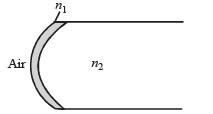Solution:

There will be no effect of the transparent thin film of uniform thickness and refractive index n1 = 1.4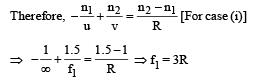∴ (a) is a correct option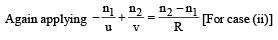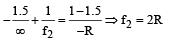∴ (c) is a correct option.

*Multiple options can be correct
QUESTION: 21

A light source, which emits two wavelength λ1 = 400 nm and λ2 = 600 nm, is used in a Young’s double slit experiment. If recorded fringe widths for λ1 and λ2 are β1 and β2 and the number of fringes for them within a distance y on one side of the central maximum are m1 and m2 respectively, then

Solution: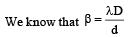As λ2 > λ1 \ β2 > β1  ∴ (a) is correct option.
Therefore m1 > m2 ∴ (b) is correct option.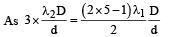3 × 600 = 4.5 × 400  ∴  (c) is correct option.

The angular width = λ/d ∴ (d) is incorrect option.

*Multiple options can be correct
QUESTION: 22

Two identical glass rods S1 and S2 (refractive index = 1.5) have one convex end of radius of curvature 10 cm. They are placed with the curved surfaces at a distance d as shown in the figure, with their axes (shown by the dashed line) aligned.
When a point source of light P is placed inside rod S1 on its axis at a distance of 50 cm from the curved face, the light rays emanating from it are found to be parallel to the axis inside S2. The distance d is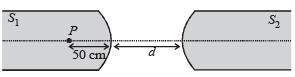Solution:

For refraction in S1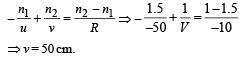For refraction in S2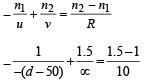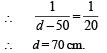B is the correct option.

*Multiple options can be correct
QUESTION: 23

A plano–convex lens is made of a material of refractive index n. When a small object is placed 30 cm away in front of the curved surface of the lens, an image of double the size of the object is produced. Due to reflection from the convex surface of the lens, another faint image is observed at a distance of 10 cm away from the lens. Which of the following statement(s) is(are) true?

Solution:

For lens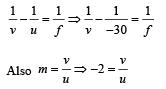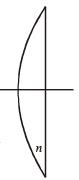On solving we get f = + 20 cm and v = 60 cm.
For reflection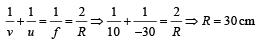The image formed by convex side is faint erect and virtual.
By lens maker formula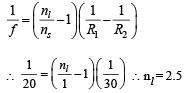*Multiple options can be correct
QUESTION: 24

A transparent slab of thickness d has a refractive index n(z) that increases with z. Here z is the vertical distance inside the slab, measured from the top. The slab is placed between two media with uniform refractive indices n1 and n2 (> n1), as shown in the figure. A ray of light is incident with angle θi, from medium 1 and emerges in medium 2 with refraction angle θt with a lateral displacement l.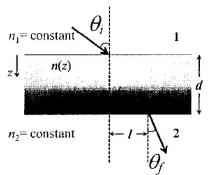Which of the following statement(s) is(are) true?

Solution:

n1 sin θi = n2 sinθf [∵ 1 and 2 interfaces are parallel] l depends on the refractive index of transparent slab but not on n2 . In fact θf depends on n2.

*Multiple options can be correct
QUESTION: 25

While conducting the Young’s double slit exper iment, a student replaced the two slits with a large opaque plate in the x-y plane containing two small holes that act as two coherent point sources (S1, S2) emitting light of wavelength 600 nm. The student mistakenly placed the screen parallel to the x-z plane (for z > 0) at a distance D = 3 m from the midpoint of S1S2, as shown schematically in the figure. The distance between the sources d = 0.6003 mm. The origin O is at the intersection of the screen and the line joining S1S2.
Which of the following is(are) true of the intensity pattern on the screen?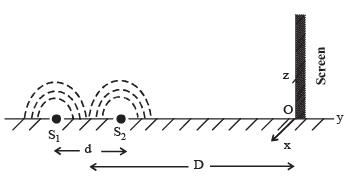Solution:

Path difference at O = d = 0.6003mm
Now, = mm = 300×10–6 mm
For n = d we get n = 2001
As n is a whole number, the condition for minima is satisfied.
Therefore ‘O’ will be dark.
Also, as the screen is perpendicular to the plane containing the slits, therefore fringes obtained will be semi-circular (Top half of the screen is available)